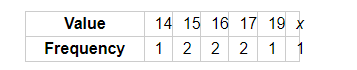# If the mode of the data: 16, 15, 17, 16, 15, x, 19, 17, 14 is 15, then x =

Question:

If the mode of the data: 16, 15, 17, 16, 15, x, 19, 17, 14 is 15, then x =

(a) 15
(b) 16
(c) 17
(d) 19

Solution:It is given that the mode of the data is 15. So, it is the observation with the maximum frequency.

This is possible only when x = 15. In this case, the frequency of 15 would be 3.

Hence, the correct answer is (a).